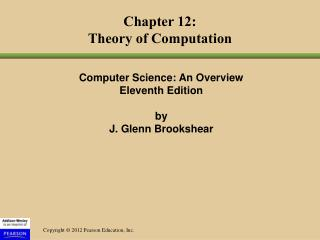DownloadDownload PresentationChapter 12: Theory of Computation

# Chapter 12: Theory of Computation

Download Presentation## Chapter 12: Theory of Computation

- - - - - - - - - - - - - - - - - - - - - - - - - - - E N D - - - - - - - - - - - - - - - - - - - - - - - - - - -
##### Presentation Transcript

1. Chapter 12:Theory of Computation Computer Science: An OverviewEleventh Edition by J. Glenn Brookshear

2. Chapter 12: Theory of Computation • 12.1 Functions and Their Computation • 12.2 Turing Machines • 12.3 Universal Programming Languages • 12.4 A Noncomputable Function • 12.5 Complexity of Problems • 12.6 Public-Key Cryptography

3. Functions • Function: A correspondence between a collection of possible input values and a collection of possible output values so that each possible input is assigned a single output

4. Functions (continued) • Computing a function:Determining the output value associated with a given set of input values • Noncomputable function:A function that cannot be computed by any algorithm

5. Figure 12.1 An attempt to display the function that converts measurements in yards into meters

6. Figure 12.2 The components of a Turing machine

7. Turing Machine Operation • Inputs at each step • State • Value at current tape position • Actions at each step • Write a value at current tape position • Move read/write head • Change state

8. Figure 12.3 A Turing machine for incrementing a value

9. Church-Turing Thesis • The functions that are computable by a Turing machine are exactly the functions that can be computed by any algorithmic means.

10. Universal Programming Language A language with which a solution to any computable function can be expressed • Examples: “Bare Bones” and most popular programming languages

11. The Bare Bones Language • Bare Bones is a simple, yet universal language. • Statements • clear name; • incr name; • decr name; • while name not 0 do; … end;

12. Figure 12.4 A Bare Bones program for computing X x Y

13. Figure 12.5 A Bare Bones implementation of the instruction “copy Today to Tomorrow”

14. The Halting Problem • Given the encoded version of any program, return 1 if the program is self-terminating, or 0 if the program is not.

15. Figure 12.6 Testing a program for self-termination

16. Complexity of Problems • Time Complexity: The number of instruction executions required • Unless otherwise noted, “complexity” means “time complexity.” • A problem is in class O(f(n)) if it can be solved by an algorithm in Q(f(n)). • A problem is in class Q(f(n)) if the best algorithm to solve it is in class Q(f(n)).

17. Figure 12.8 A procedure MergeLists for merging two lists

18. Figure 12.9 The merge sort algorithm implemented as a procedure MergeSort

19. Figure 12.10 The hierarchy of problems generated by the merge sort algorithm

20. Figure 12.11 Graphs of the mathematical expressions n, lg n, n lg n, and n2

21. P versus NP • Class P: All problems in any class Q(f(n)), where f(n) is a polynomial • Class NP: All problems that can be solved by a nondeterministic algorithm in polynomial time Nondeterministic algorithm = an “algorithm” whose steps may not be uniquely and completely determined by the process state • Whether the class NP is bigger than class P is currently unknown.

22. Figure 12.12 A graphic summation of the problem classification

23. Public-Key Cryptography • Key: A value used to encrypt or decrypt a message • Public key: Used to encrypt messages • Private key: Used to decrypt messages • RSA: A popular public key cryptographic algorithm • Relies on the (presumed) intractability of the problem of factoring large numbers

24. Encrypting the Message 10111 • Encrypting keys: n = 91 and e = 5 • 10111two = 23ten • 23e = 235 = 6,436,343 • 6,436,343 ÷ 91 has a remainder of 4 • 4ten = 100two • Therefore, encrypted version of 10111 is 100.

25. Decrypting the Message 100 • Decrypting keys: d = 29, n = 91 • 100two = 4ten • 4d = 429 = 288,230,376,151,711,744 • 288,230,376,151,711,744 ÷ 91 has a remainder of 23 • 23ten = 10111two • Therefore, decrypted version of 100 is 10111.

26. Figure 12.13 Public key cryptography